1. ##linking dots with the Aztec Stone Calender...

looking at the aztec calender brings up some interesting results re geometry of the sacred kind....then again isnt all geometry sacred?!.....anyway...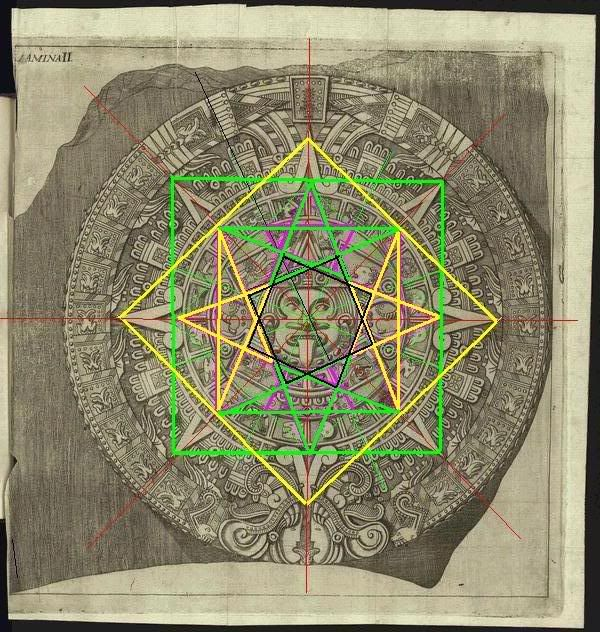the 4 5 pointed stars around the central face is nice, i wonder if that was a part of the original template for the carving? not surprising tho given venus is a vital part of mayan culture so lets assume lines were drawn when it was layed out before carving....OR...is it like a lot of these artifacts, hidden messages or symbols of a multi dimensional nature!

also in black are 2 offset squares which are both at 60 degrees to the vertical = 120 degrees

the stone calendar has a diameter of exactly 3.6 metres. but in feet is 11.8ft x modern pi = circumference of 37.07ft obviously very close to the factor of 37 and also converts to 444 inches

the factor 37 and the Great Pyramid (GP) are connected to the stone calendar ie: 481ft height/37ft = 13 and 756ft one side/37ft = 20.43 which is 20 & 13 the mayan calendar round of 13 months of 20 days the tzolkin 260 day count. Also the length of the GP base is 3024ft/37 = 81.729 which reminds me of the ratio of 1/81 = 0.0123456790 which adds up to ...you guessed it 37!

the penta-stars around the centre face could well symbolise Venus rounds which as you know is 584 days. in Harmonic octave terms if we go down three octves 584/292/146/73 we get a mirror version of 37. And, oddly 37 and 73 are primes 12 and 21

73/37 = 1.972972r -1.0 this is a harmonic of th earth grid of 97,200 arc seconds (which is 27hrs not our usual 24hrs)

here's an image with just the lines and 'Iron cross' added where the green and yellow squares meet: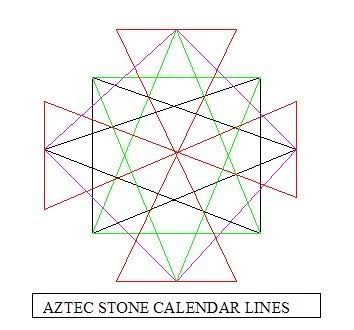you can see other archetypes in this too, like the six pointed "Star of Solomon"..and indeed the ''Merkabah''

http://www.paleoanimation.us/animation.html

SUPRISE this may not, but the aztec stone calendar star is identical to that of the Washington DC configuration giving the impression that time/geometry/space at all one and it is there reflected in the physical arcane knowledge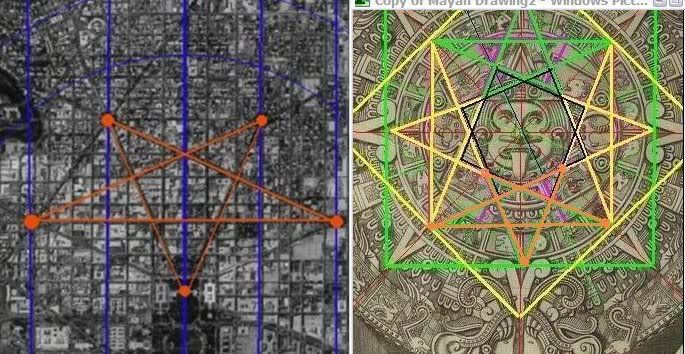if there is any great failing in mainstream science it is the lack of attention re the power of geometry....

peace always
mike

tbc..

¤=[Post Update]=¤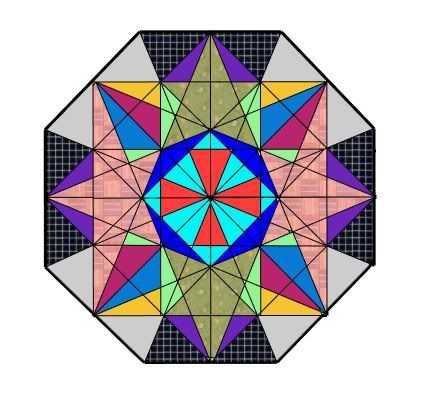using sketchup other correlations can be seen to the cubic cross and the face flattened cube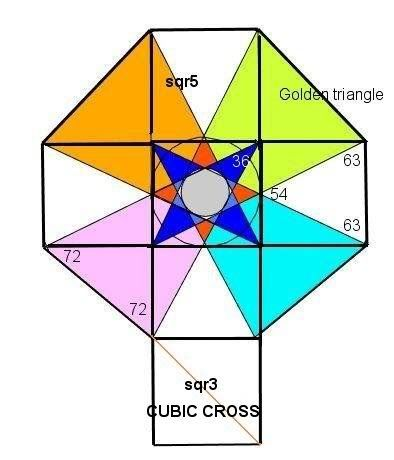the flattened cube has hidden golden triangles based on the diagonals [square root of 5]. also the almost equilateral triangles of 54 and 63 degrees (4 x 54 =216) (63 x 8 =504) note 5040 is Earth/Moon radius 

i wonder just how many churches are designed upon the cube and how many Egyptian deities sit on a cube throne??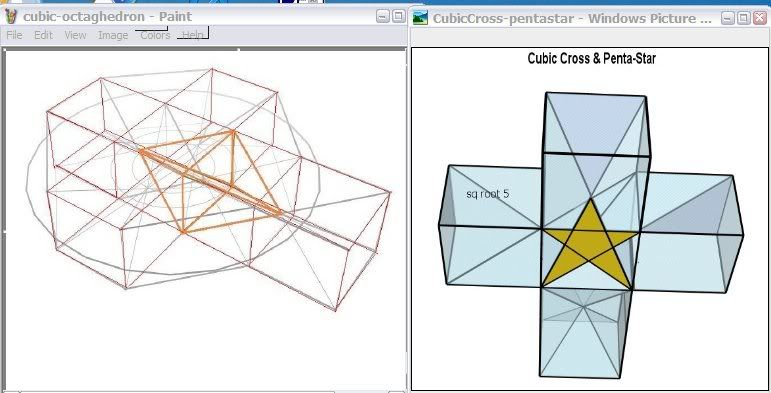....an example of the cubic representation in the layout of the Florence Duomo Cathedral note the 'key' theme - same as St Peter's Basilica to name just a couple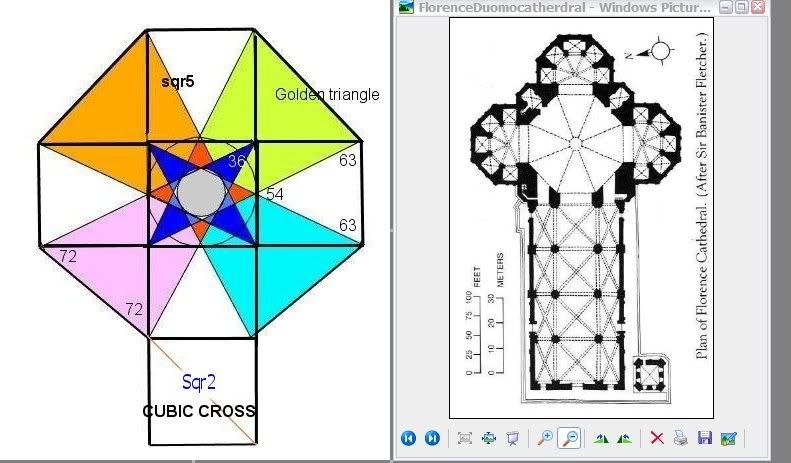interesting...

peace always
mike

tbc..Reply With Quote

2. ##Re: linking dots with the Aztec Stone Calender...

..in refining the mandala at a lower level we find some interesting harmonics:

the pattern that emerges from this image is the eight pointed cross also known as the Maltese cross of the Knights of St John.....but one feels it had earlier connections...probably masonic...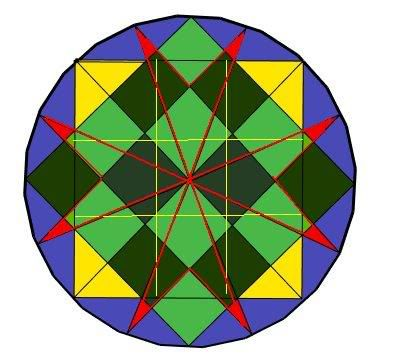in the pattern you can see two opposing squares, they lay the pattern for the cross

once the arms of the cross are complete we have a yellow square with 9 square grid, which is overlayed with another square grid of 16

the result is 9 x 16 = 144 (gematria for light) a very harmonic number. note 3 squared and 4 squared = 7 squared (sacred number)

the results if one is looking: 49-9 = 40 (the bible is full of 40s) 49-16 = 33rd degree mason 24hrs + 16 + 9 = 49

the angles of the cross at the centre are 45 x 4 = 180 and each triangle formed at the base is 67.5 x 8 = 540 540/180 = 3 the trinity. But 67.5 is an excellent harmonic see further:

while 540+180 = 720degrees x 60min x 60sec = 25920{0} precessional cycle

also it hides the nature's number '5' in 9+16 = 25 (5 squared)

so now we come to the basis of this pattern [squared]

9-16-25 (symbolically Pythagorus 3-4-5 triangles the basis of masonic geometry and music

the 2 squares extended as the 8 pointed cross become 22.50 degrees in circle. 22.5 x 60min x 60sec = 81,000 or 81 (9x9) In masonic rituals I read of a ceremony to the temple of Solomon and lighting of 81 candles (lodge draped in black) and in the fourth degree the ritual is the decoration of the lodge in green and lighting of sixteen candles. (read the green as the 5th chakra and fourth colour)

67.5/135/270/540/1080/2160/432/864 (4 Octaves up = Moon) (5 Octaves up = 1/12 zodiac) (7 Octaves up = Sun)

Nineveh cycle 1959552/81 = 24192
24192/12096/6048/3024 (3 octaves down) perimeter of base of Great Pyramid (GP)

Nineveh 1959552/67.5 = 29030.40 doesn't look like much until 3 octaves down 3628.80 GP base 3024ft x 12 = 36,288 inches or 1to 9 multiplied is 362,880 or approx GP height 480ft x side base 756ft = 362,880

which is = 8 rounds of Saturn cycle @ 378days

so the above geometric mandala is proving to be much more than a symbol of protection. it's a symbol of cosmic unity or order via geomtery and harmonics

...further on...you can see that from two opposing squares (or bases of a pyramid - perhaps why the GP has indents half way along the sides) inscribed in the circle hides more than the Maltese cross. There is also the seal of Solomon, which fits within the geometry to code various knowledge of cycles etc - http://www.code144.com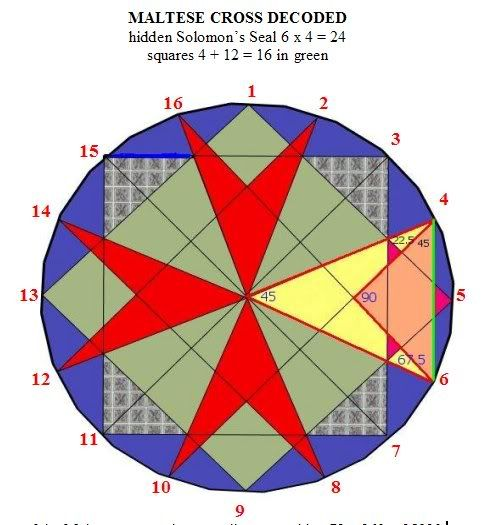numbers of the Maltese cross points are all even - add to 72 x 360 = 25920 precession. While the odd numbers of the two squares total to 64

Square 1 = 28
Square 2 = 36

apex triangles 45:90 = 1:2 360/8 = 45
bases 22.5:45 = 1:2 360/16 = 22.5
bases 22.5:67.5 = 1:3

note: min sec are arcs

360/22.5 = 16 degrees x 60min x 60sec = 57600
Harmonics: 6 octaves â 288/144/72/36/18/9

22.5 degrees x 60min x 60sec = 81,000 x 8 = 648,000
Harmonics: 648 octaves á 1296/2592 precessional cycle

45 degrees x 60min x 60sec = 162,000 (exact speed of light in nautical mps)
Harmonics: 162 x 8 = 1296 (half precession)

Nineveh cycle 195955200000000/67.5 degrees = 2903040000000
Harmonics: 3 Octaves â 2903040/1451520/725760/362880 numbers 1 to 9 multiplied or Great Pyramid 480ft x 756ft = 362880 or perimeter of base 3024ft = 36288inches. 6 octaves â 36288/18144/9072/4536 [453.6ft truncated height of GP)
in Bruce Cathies harmonic grid work he uses 97200 grid sec or 27hrs

in the above 16 x 27 = 432

[432x432] is speed of light within official speed mps of 0.0015%

195955200000000 / 186,624= 105,0000000 /60sec/60min

291666.66666666666666666666666666hrs x 24 = 7,000,000 days
7,000,000 days/365.242 = 19165.375
Harmonics: Lunar Metonic cycle: 19.1 years

This is an amazing harmonic result that the cycle of the galaxy converted to light mps is exactly 7,000,000. That the Nineveh cycle against the light harmonic is also 105 (3x5x7)
and Polygonal number stacking of spheres [Dodecagonal that include both 64 and 105]

interesting...

peace always
mike

tbc..Reply With Quote

3. ##Re: linking dots with the Aztec Stone Calender...

...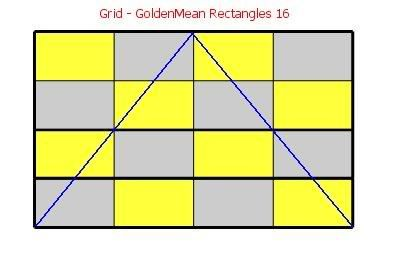the above grid is based on one major golden rectangle with 16 stacked within it (using sketchup again!)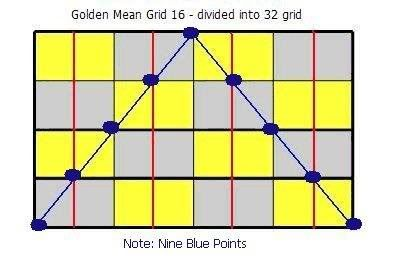..a triangle is drawn from the base corners to top centre point.
then where the triangle intersects the rectangles a further grid is created totalling 32 rectangles (not squares)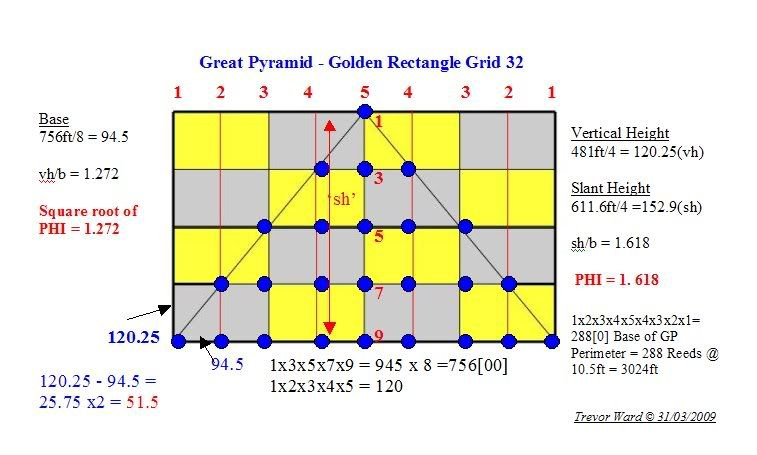there are 45 intersection points in the grid and the triangle has 25 points. Note there’s 10 points each side outside the triangle. 25/45 = 0.555

this is quite amazing IMHO, the distribution of intersecting points of 45/20/25 is on a ratio of 9:4:5

the single digit numbers of the points show the relationship of the vertical 1-3-5-7-9 to the base of the Great Pyramid and that the horizontal points have half of the points 1x2x3x4x5 within 0.25 of the base height 481ft/4

the base side of 756ft is divided into 8 units, while the height into 4 units as per the grid. Resulting in the Great Pyramid measurements yielding PHI and square root of PHI

a quick search on google to find similar info and only found the following example which dovetails beautifully with the previous posts on the Maltese cross and its appearance in the geometry from egyptian and aztec cultures -

"The above value of Pi was later confirmed by F Gaendiger, who ingeniously deduced it from the well-known grid technique that the ancient Egyptians used as a background for their drawings. By imagining a grid of 10 x 10 squares, each measuring 1c x 1c, then by drawing a circle within the grid, the radius of the circle will measure 5c. The periphery of the circle will pass by 12 points of the grid; 4 points are given by the axes, while 8 further points are given by eight triangles measuring 3-4-5 cubits (arranged in the form of a Maltese cross). The 12 points divide the periphery of the circle into 4 short and 8 long arcs. By measuring these we find simple numbers: the long arc = 90 digits (28 digits make 1 cubit) and the small arc = 40 digits (or a few mms less). Hence the circumference of the circle measures 4x40 + 8x90 = 880 digits = 220/7 cubits. Dividing this measurement by the diameter 10 cubits gives exactly Pi = 22/7." ref:
http://www.freerepublic.com/focus/f-news/2025333/posts
http://weekly.ahram.org.eg/2008/890/heritage.htm

in fact the Maltese cross referred to here to is really an Iron Cross (Red/Green)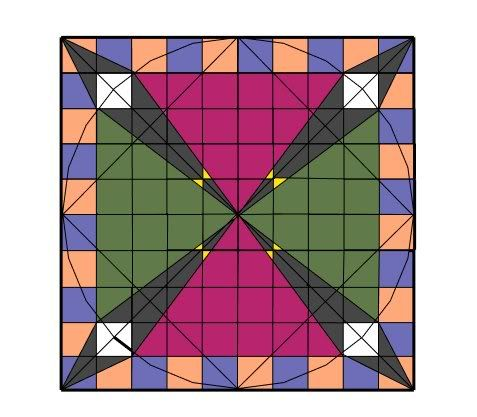the Great Pyramid "missing" capstone that was to be put in place atop the GP in the new Millenium was reported to be 13.5ft at base and 8.58 ft high. 13.5ft x7 = 94.5ft. The height is supposed to be 1/56th of the height of the GP 8.589 and 8.589 x 14 =120.25. a perfect marriage of the numbers in the grid - http://www.geocities.com/davidjayjordan ... d1-56.html

im not sure what to make of it all but its interesting stuff...

peace always
mike

(rant over)Reply With Quote

####Posting Permissions

• You may not post new threads
• You may not post replies
• You may not post attachments
• You may not edit your posts